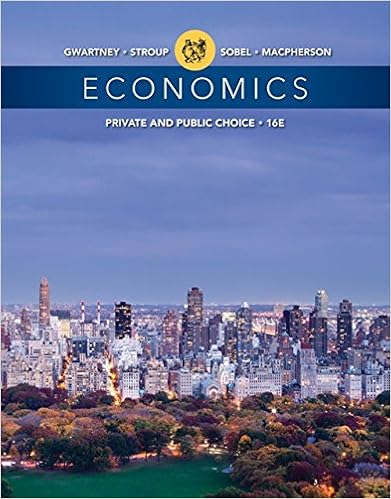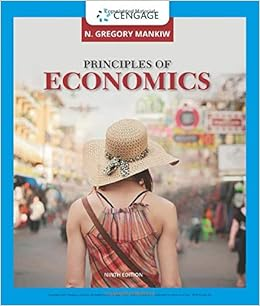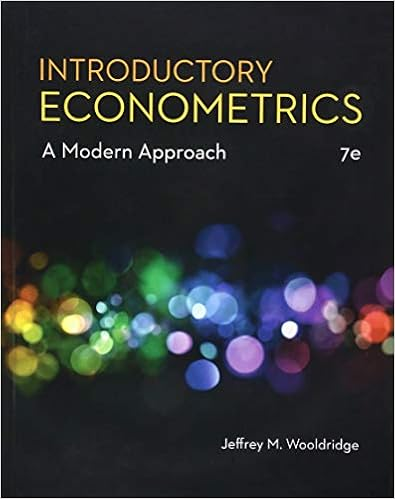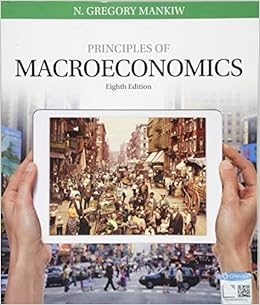# W3213_Final_2010 - Columbia University Final Exam...

• Test Prep
• 3
• 86% (14) 12 out of 14 people found this document helpful

This preview shows page 1 - 3 out of 3 pages.

Columbia University Final ExamIntermediate Macroeconomics W3213Fall 2010 (A.D.)Professor XavierANSWER ALL four QUESTIONS.ANSWER EACH QUESTION IN A SEPARATE BLUE BOOK.NOTE: YOU ARE NOT ALLOWED TO USE CALCULATORS!!!!Question 1.-­૒ Short Speeches(a)Because ideas are “non-­૒rival” goods, inventors have little economic incentives to inventsince they cannot prevent others from copying their ideas. Do you agree? Discuss.(b)In the real world there is evidence that economies tend to “convergeconditionally” (thatis, there is“conditional convergence”) but not that they tend to “converge absolutely”(that is, there is no “absolute convergence”).(c)In the Keynesian model, a sudden increase in money DEMAND causes a recession. Doyou agree? Explain using the IS/LM model(d)Imagine that, instead of using the traditional assumption that “output is demanddetermined” we assume that “output is determined by the short side of the market”(that is, for interest rates for which supply is smaller than demand, output isdetermined by supply; and for interest rates for which demand is smaller than supply,output is determined by demand). Starting from a position of equilibrium betweendemand and supply, what would be the effects of an increase in money supply?Question 2.-­૒ Productivity GrowthAssume that an economy has the production function Y = K1/3L1/3T1/3, where K is capital, Lis labor, and T is land.(a)Show that this function exhibits constant returns to scale.(b)Is the return to capital diminishing? Show why.(c)State the production function per worker.For this economy, the saving rate is equal to 1/3 and the depreciation rate is equal to 1/12.There is neither population growth nor technological growth. The amount of land perworker is equal to 1 and the amount of capital per worker is 8.(d)Write down the fundamental equation of Solow-­૒Swan with this production function.(e)What is the growth rate of capital per person? Where is this economy on the graph?(f)What is the long-­૒run rate of output growth in the economy? Why?Imagine that 3/4 of its land became permanently flooded. Luckily, the flood was predictedwell in advance so no people died and all capital was saved despite the disaster. Assumethat the economy was at its steady state before the flood.
##### We have textbook solutions for you!The document you are viewing contains questions related to this textbook.
Chapter 14 / Exercise 7
Economics: Private and Public Choice
Gwartney/Stroup/Sobel/MacphersonExpert VerifiedBrowse all Textbook Solutions
(g)Explain what happens to capital per capita and output per capita immediately after theflood. What happens during the transition to the new steady state? (qualitative answeris sufficient)(h)To prevent capital from declining, the government proposes to stimulate saving in theeconomy. What would be the result of this action in the short run? In the long run? Isthis a good idea?Question 3.-­૒ Solow-­૒SwanThe production function for an economy is Yt= 1.5 KtαLt(1-­૒α), where Ytis total output, Ktistotal capital, and Ltis total labor. Total savings for the economy is given by St= 0.2 Yt. Therate of population growth,n, is 5% and the rate of depreciation, δ, is 10%.(a)Write down the per capita production function.(b)Write down the fundamental equation of Solow-­૒Swan.(c)Imagine that α=0.7. In the long run, what is the growth rate of per capita output, yt?In the long run, what is the growth rate of total output, Yt?(d)In the long run, is there a constant level of capital per person, output per person andconsumption per person? If so what are they? If not, why not?(e)Imagine that the depreciation rate increases from 10% to 12%. If the economy isinitially in steady state, what are the effects on the levels and growth rates of k, y, and c?(f)Imagine that α=1.
End of preview. Want to read all 3 pages?

Upload your study docs or become a

Course Hero member to access this document

Term
Fall
Professor
XAVIER
Tags
Per capita, interest rates
##### We have textbook solutions for you!
The document you are viewing contains questions related to this textbook.The document you are viewing contains questions related to this textbook.
Chapter 14 / Exercise 7
Economics: Private and Public Choice
Gwartney/Stroup/Sobel/MacphersonExpert Verified
•••